## Data-Communication

 Question 1
The resistance to be connected across terminal a, b for maximum power transfer to it is: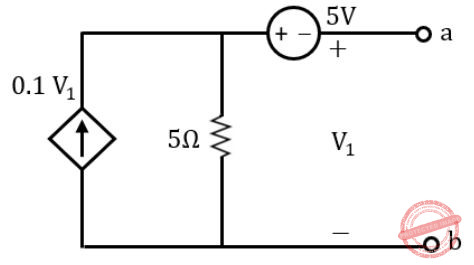A 40Ω B 5Ω C 2.5Ω D 10Ω
Data-Communication       Resisters       NIC-NIELIT Scientist-B 2020
 Question 2
A special PCM system uses 32 channels of data, one whose purpose is an identification (ID) and synchronization. The sampling rate is 4 kHz. The word length is 5 bits. Find the serial data rate.
 A 1280 kHz B 160 kHz C 320 kHz D 640 kHz
Data-Communication       PCM       NIC-NIELIT Scientist-B 2020       Video-Explanation
 Question 3
Calculate the modulation percentage if the modulating signal is 8 V and carrier is of 12 V?
 A 50 B 67 C 150 D 33
Data-Communication       Modulation-Index       NIC-NIELIT Scientist-B 2020       Video-Explanation
Question 3 Explanation:
Modulation index(m) = Vm/Vc
= (8/12)*100
= 66.66.
 Question 4
What is the basis of KVL?
 A Conservation of charge B Conservation of energy C Conservation of power D All of the options
Data-Communication       KVL       NIC-NIELIT Scientist-B 2020       Video-Explanation
Question 4 Explanation:
Kirchhoff’s Voltage Law
Kirchhoff’s Voltage Law (KVL) is Kirchhoff’s second law that deals with the conservation of energy around a closed circuit path.Question 5
In which modulation discrete values of carrier frequencies is used to transmit binary data?
 A Phase Shift Keying B Amplitude Shift Keying C Frequency Shift Keying D Disk Shift Keying
Data-Communication       Frequency-Shift-Keying       NIC-NIELIT Scientist-B 2020       Video-Explanation
Question 5 Explanation:
Frequency-shift keying (FSK) is a frequency modulation scheme in which digital information is transmitted through discrete frequency changes of a carrier signal. The technology is used for communication systems such as telemetry, weather balloon radiosondes, caller ID, garage door openers, and low frequency radio transmission in the VLF and ELF bands. The simplest FSK is binary FSK (BFSK). BFSK uses a pair of discrete frequencies to transmit binary (0s and 1s) information. With this scheme, the “1” is called the mark frequency and the “0” is called the space frequency.
 Question 6
The period of a signal is 100ms. then the frequency of this signal in kilohertz is ____?
 A 10 B 10-1 C 10-2 D 10-3
Data-Communication       Signal       UGC NET JRF November 2020 Paper-2
Question 6 Explanation:
T=100ms
= (100)10-3 sec
=10-1 sec
F=1/T
F=1/10-1 Hz
F=1/(10-1 *103) KHz
F = 10-2 KHz
 Question 7
ECL is the fastest of all logic families. High speed in ECL is possible because transistors are used in difference amplifier configuration, in which they are never driven into ____.
 A Race condition B Saturation C Delay D High impedance
Data-Communication       ideal-op-amp       UGC NET CS 2017 Jan -paper-2
Question 7 Explanation:
→ ECL is the fastest of all logic families. High speed in ECL is possible because transistors are used in difference amplifier configuration, in which they are never driven into Saturation.
→ Emitter-coupled logic (ECL) is a high-speed integrated circuit bipolar transistor logic family. ECL uses an overdriven BJT differential amplifier with single-ended input and limited emitter current to avoid the saturated (fully on) region of operation and its slow turn-off behavior.
→ As the current is steered between two legs of an emitter-coupled pair, ECL is sometimes called current-steering logic (CSL), current-mode logic (CML) or current-switch emitter-follower (CSEF) logic.[
 Question 8
Which of the following is/are true w.r.t. applications of mobile computing ?
(A) Travelling of salesman
(B) Location awareness services
 A (A) true; (B) false. B Both (A) and (B) are true. C Both (A) and (B) are false. D (A) false; (B) true.
Data-Communication       Mobile-Computing       UGC NET CS 2017 Jan -paper-2
Question 8 Explanation:
Mobile computing main applications:
1.Portability
2. Connectivity
3.Social Interactivity
4. Individuality
5. IMobility
Travelling of salesman applicable to mobility of application.
Location awareness services applicable to Connectivity.
 Question 9
The frequency band allocated for the downlink in GSM is :
 A 960 – 985 MHz B 935 – 960 MHz C 920 – 945 MHz D 930 – 955 MHz
Data-Communication       Cellular-Networks       UGC NET CS 2006 Dec-paper-2
Question 9 Explanation:
There are 2 popular GSM methods.
1. GSM-900
2. GSM-1800
GSM-900 uses 890 – 915 MHz to send information from the Mobile Station to the Base
Transceiver Station (uplink) and 935 – 960 MHz for the other direction (downlink), providing 124 RF channels (channel numbers 1 to 124) spaced at 200 kHz. Duplex spacing of 45 MHz is used.
 Question 10
The frequency reuse plan is divided into cell grouping using how many cells, where the number of cells equals N ?
 A 3 B 10 C 7 D 21
Data-Communication       Cellular-Networks       UGC NET CS 2005 june-paper-2
Question 10 Explanation:
The cluster size N = 7 and the frequency reuse factor is 1/7 since each cell contains one-seventh of the total number of available channels.
Total cells=7*3
=21Frequency reuse concept, cells with the same letter use the same set of frequencies. A cell cluster is outline in blue color and replicated over the converge area.
 Question 11
Which interim standard describes inter-switching networking ?
 A IS – 54 B IS – 95 C DS – 45 D ANSI – 41
Data-Communication       Cellular-Networks       UGC NET CS 2005 june-paper-2
Question 11 Explanation:
→ Interim Standard 95 (IS-95) was the first ever CDMA-based digital cellular technology.
→ The IS-95 standards describe an air interface, a set of protocols used between mobile units and the network.
→ IS-95 is widely described as a three-layer stack, where L1 corresponds to the physical (PHY) layer, L2 refers to the Media Access Control (MAC) and Link-Access Control (LAC) sublayers, and L3 to the call-processing state machine.
 Question 12
Theoretically, how many 1.25 MHz Carriers are there in a CDMA cell ?
 A 18 B 22 C 9 D 64
Data-Communication       Cellular-Networks       UGC NET CS 2005 june-paper-2
Question 12 Explanation:
→ In CDMA, multiple users share a 1.25 MHz channel by using orthogonal spreading codes (Walsh codes)
→ IS-95a standard designed for AMPS cellular band
→ Each cellular provider is allocated 25 MHz spectrum and ten 1.25-MHz CDMA duplex channels if A AMPS Band provider, 9 if B band provider
 Question 13
The mechanism with which several uses can share a medium without interference is :
 A Frequency modulation B Amplitude modulation C Multiplexing D None of these
Data-Communication       Multiplexing        UGC NET CS 2005 Dec-Paper-2
Question 13 Explanation:
Multiplexing is a method by which multiple analog or digital signals are combined into one signal over a shared medium. It can share a medium without interference.
 Question 14
Which of the following services is not provided by wireless access point in 802.11 WLAN ?
 A Association B Disassociation C Error correction D Integration
Data-Communication       WLAN       UGC NET CS 2015 Dec- paper-2
Question 14 Explanation:
→ Wireless LANs are use high frequency radio waves instead of cables for connecting the devices in LAN. Users connected by WLANs can move around within the area of network coverage. Most WLANs are based upon the standard IEEE 802.11 or WiFi.
→ A wireless access point (WAP), or more generally just access point (AP), is a networking hardware device that allows other Wi-Fi devices to connect to a wired network.
→ Wireless access point in 802.11 WLAN services are association, association and Integration.
 Question 15
Four channels are multiplexed using TDM. If each channel sends 100 bytes/second and we multiplex 1 byte per channel, then the bit rate for the link is __________.
 A 400 bps B 800 bps C 1600 bps D 3200 bps
Data-Communication       Multiplexing        UGC NET CS 2015 Dec- paper-2
Question 15 Explanation:
Given data,
— 4 channels are multiplexed using TDM
— Multiplex 1 byte per channel. So, total 4 bytes=4 channels.
— Each channel sends 100 bytes/sec
— Bit rate=?
Bit rate= 100 * 4 bytes(1 byte=8 bits)
= 100 * 4 *8 (we are converting into bits because we are finding bit rate)
= 3200 bps
 Question 16
In a typical mobile phone system with hexagonal cells, it is forbidden to reuse a frequency band in adjacent cells. If 840 frequencies are available, how many can be used in a given cell?
 A 280 B 110 C 140 D 120
Data-Communication       Frequency-Band       UGC NET CS 2015 Dec- paper-2
Question 16 Explanation:
— Mobile phone system with hexagonal( equals to 6) cells
— 840 frequencies are available.
— How many can be used in a cell?
In this we need maximum 3 unique cells are required.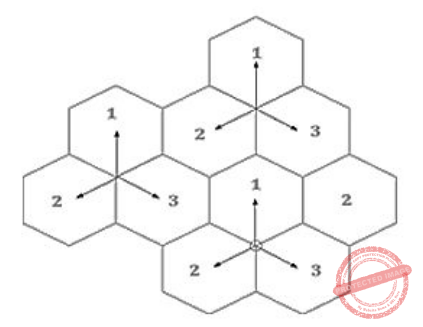Total cells used with frequency is = 840/3
= 280 frequencies
 Question 17
A data cube C, has n dimensions, and each dimension has exactly p distinct values in the base cuboid. Assume that there are no concept hierarchies associated with the dimensions. What is the maximum number of cells possible in the data cube, C?
 A p​ n B p C (2​ n​ – 1)p+1 D (p + 1)​ n
Data-Communication       Mobile-Computing       UGC NET CS 2015 Dec- paper-2
Question 17 Explanation:
Option-A:​ This is the maximum number of distinct tuples that you can form with p distinct values per dimensions.
Option-B:​ You need at least p tuples to contain p distinct values per dimension. In this case no tuple shares any value on any dimension.
Option-C:​ The minimum number of cells is when each cuboid contains only p cells, except for the apex, which contains a single cell.
Option-D:​ We have p+1 because in addition to the p distinct values of each dimension we can also choose ∗.
 Question 18
The _____ measures the relative strengths of two signals or a signal at two different points
 A frequency B decibel C Attenuation D throughput
Data-Communication       Signal       KVS 30-12-2018 Part B
Question 18 Explanation:
Decibel (dB), unit for expressing the ratio between two physical quantities, usually amounts of acoustic or electric power, or for measuring the relative loudness of sounds. One decibel (0.1 bel) equals 10 times the common logarithm of the power ratio
 Question 19
The process of converting the analog sample into discrete form is called
 A Modulation B Multiplexing C Quantization D Sampling
Data-Communication       Sampling-Types       Nielit Scientist-B CS 22-07-2017
Question 19 Explanation:
Sampling is the process of recording an analog signal at regular discrete moments of time.
sampling is the reduction of a continuous-time signal to a discrete-time signal. A common example is the conversion of a sound wave (a continuous signal) to a sequence of samples (a discrete-time signal).
Quantization in digital signal processing, is the process of mapping input values from a large set (often a continuous set) to output values in a (countable) smaller set, often with a finite number of elements.
The sampling rate fs is the number of samples per second.
The time interval between samples is called the sampling interval Ts=1/fs
 Question 20
A stable multivibrator are used as
 A comparator circuit B squaring circuit C frequency to voltage converter D voltage to frequency converter
Data-Communication       stable-multivibrator       Nielit Scientist-C 2016 march
Question 20 Explanation:
● A multivibrator is a one type of electronic circuit, that is used to implement a two state system like flip-flops, timers and oscillators.
● Multivibrators are classified into three types based on the circuit operation, namely Astable multivibrators, Bistable multivibrators and Monostable multivibrators.
● The astable multivibrator is not stable and it repeatedly switches from one state to the other. In monostable multivibrator, one state is stable and remaining state is unstable.
● When the power is turned ON consider the flip flop is cleared initially, then the o/p of the inverter will be high. The charging of the capacitor will be done using two resistors R1& R2. When the voltage of the capacitor goes above 2/3 Vcc, then the output of the higher comparator will be High, it changes the control flip flop
 Question 21
The astable multivibrator has
 A two quasi stable states B two stable states C one stable and one quasi-stable state D none of these
Data-Communication       stable-multivibrator       Nielit Scientist-C 2016 march
Question 21 Explanation:
A multivibrator is an electronic circuit used to implement a variety of simple two-state devices such as relaxation oscillators, timers and flip-flops. It consists of two amplifying devices (transistors, vacuum tubes or other devices) cross-coupled by resistors or capacitors.
 Question 22
Which of the following is not a transceiver function?
 A Transmission and receipt of data B Checking of line voltages C Addition and subtraction of headers D Collision detection
Data-Communication       Transceiver       Nielit Scientist-B CS 2016 march
Question 22 Explanation:
A transceiver is a device comprising both a transmitter and a receiver that are combined and share common circuitry or a single housing. When no circuitry is common between transmit and receive functions, the device is a transmitter receiver.
 Question 23
Vestigial sideband most commonly used in radio transmission
 A radio transmission B telephony C television transmission D all of the above
Data-Communication       Radio-Transmission       NieLit STA 2016 March 2016
Question 23 Explanation:
Limitation of single-sideband modulation being used for voice signals and not available for video/TV signals leads to the usage of vestigial sideband. A vestigial sideband (in radio communication) is a sideband that has been only partly cut off or suppressed. Television broadcasts (in analog video formats) use this method if the video is transmitted in AM, due to the large bandwidth used. It may also be used in digital transmission, such as the ATSC standardized 8VSB.
Vestigial sideband (VSB) is a type of amplitude modulation ( AM ) technique (sometimes called VSB-AM ) that encodes data by varying the amplitude of a single carrier frequency . Portions of one of the redundant sidebands are removed to form a vestigial sideband signal – so-called because a vestige of the sideband remains.
 Question 24
When the object making the sound is moving towards you, the frequency goes up due to he waves getting pushed more tightly together. The opposite happens when the object moves away from you and the pich goes down. This phenomenon is called
 A Band Width B Doppler effect C Sound refraction D Vibrations
Data-Communication       Doppler-Effect       KVS DEC-2013
Question 24 Explanation:
→ The Doppler effect is the change in frequency or wavelength of a wave in relation to an observer who is moving relative to the wave source.
→ The Doppler effect is observed whenever the source of waves is moving with respect to an observer.
→ The Doppler effect can be described as the effect produced by a moving source of waves in which there is an apparent upward shift in frequency for observers towards whom the source is approaching and an apparent downward shift in frequency for observers from whom the source is receding.
 Question 25
The closeness of the recorded version to the original sound is called
 A Fidelity B Digitization C Sampling D Nyquist Theorem
Data-Communication       Fidelity       KVS DEC-2013
Question 25 Explanation:
→ Digitization is the process of converting information into a digital format . In this format, information is organized into discrete units of data (called bit s) that can be separately addressed (usually in multiple-bit groups called bytes).
→ sampling is the reduction of a continuous-time signal to a discrete-time signal. A common example is the conversion of a sound wave (a continuous signal) to a sequence of samples (a discrete-time signal).
→ “fidelity” denotes how accurately a copy reproduces its source
 Question 26
The diagram below represents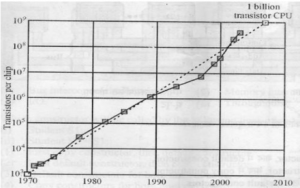A Moore’s law B Newton raphson method C Boyle’s law D Gregor law
Data-Communication       Moore’s-law       KVS DEC-2013
Question 26 Explanation:
Moore’s law is the observation that the number of transistors in a dense integrated circuit doubles about every two years.
 Question 27
A___ multivibrator circuit is one in which both LOW and HIGH output states are stable
 A Monostable B Bistable C Multistable D Tristable
Data-Communication       stable-multivibrator       KVS DEC-2013
Question 27 Explanation:
● The Bistable Multivibrator is another type of two state device.
● Bistable Multivibrators have TWO stable states (hence the name: “Bi” meaning two) and maintain a given output state indefinitely unless an external trigger is applied forcing it to change state.
 Question 28
Refer to the monostable multibrator circuit in the figure below.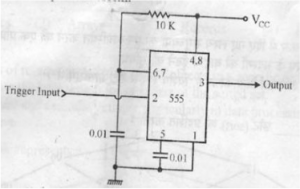The trigger terminal(pin 2 of the IC) is driven by a symmetrical pulsed waveform of 10kHz. Determine the duty cycle of the output waveform.
 A 0.56 B 0.55 C 0.57 D 0.58
Data-Communication       stable-multivibrator       KVS DEC-2013
Question 28 Explanation: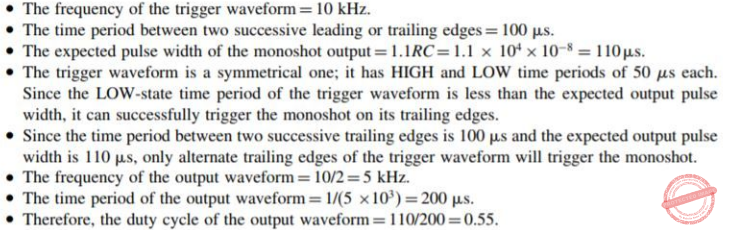Question 29
The percentage resolution of an eight bit D/A converter is
 A 0.39% B 0.38% C 0.50% D 0.51%
Data-Communication       A/D-Converter       KVS DEC-2013
Question 29 Explanation:
→ An eight-bit D/A converter have 2​ 8​ -1 resolvable levels which is nothing but 255 levels.
→ Percentage resolution is (1/255)×100=0.39%
 Question 30
The diagram below depicts:A Sound in waveform B Wind frequency C Line of sight D Compressions on a map
Data-Communication       Waveform       KVS DEC-2013
Question 30 Explanation:
●The generic term waveform means a graphical representation of the shape and form of a signal moving in a gaseous, liquid, or solid medium.
●For sound, the term describes a depiction of the pattern of sound pressure variation (or amplitude) in the time domain
● The temporal frequencies of sound waves are generally expressed in terms of cycles (or kilocycles) per second. The simplest waveform is the sine wave, since it has only one frequency associated with it. The sound waves associated with, say, music, are constantly varying.
 Question 31
The diagram below represents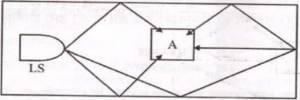A Resolution B Vibration C Reverberation D Frequency
Data-Communication       Reverberation       KVS DEC-2013
Question 31 Explanation:
● Reverberation, in psychoacoustics and acoustics, is a persistence of sound after the sound is produced.
● A reverberation, or reverb, is created when a sound or signal is reflected causing a large number of reflections to build up and then decay as the sound is absorbed by the surfaces of objects in the space – which could include furniture, people, and air.
 Question 32
The process of taking a snapshot of the waveform at regular intervals and representing it as a binary number is known as
 A Sampling B Standard Assessment C Sequential Formatting D Sound Structure
Data-Communication       Digital-sampling       KVS DEC-2013
Question 32 Explanation:
Digital sampling is the recording of a sound using a string of numbers to represent the sound. 1s and 0s. These numbers are called samples.
 Question 33
In MOSFET fabrication, the channel length is delayed during the process of
 A Isolation oxide growth B Channel stop implantation C Poly-Silicon gate patterning D Lithography step leading to the contact pad
Data-Communication       MOSFET       Nielit Scientific Assistance CS 15-10-2017
Question 33 Explanation:
In MOSFET fabrication channel length is defined during Poly-Silicon gate patterning process
 Question 34
​ An ideal op-amp is an ideal
 A Voltage controlled current source B voltage controlled voltage source C current controlled current source D current controlled voltage source
Data-Communication       ideal-op-amideal-op-amp       Nielit Scientific Assistance IT 15-10-2017
Question 34 Explanation:
An ideal op-amp lead us to an op-amp with input resistance is infinite, so no current flows into either input terminal (the “current rule”) and that the differential input offset voltage is zero (the “voltage rule”).
Ideal operational amplification will have
➝ High input impedance ( R in = ∞ ) and low output impedance ( R out = 0 )➝ R in = ∞ , so zero input current
➝ Infinite voltage gain
➝ So, it is a voltage controlled voltage source device.
 Question 35
A carrier A​ c​ Cos(w​ C​ )t is frequency modulated by a signal E​ m​ Cos(w​ m​ )t. The modulation index is m​ f​ . the expression for the resulting FM signal is
 A A​ c,​ Cos[w​ c​ t+ m​ f​ Sin(w​ m​ )t] B A​ c​ Cos[w​ c​ t+ m​ f​ Cos(w​ m​ )t] C A​ c​ Cos[w​ c​ t+ ​ π ​ m​ f​ Sin w​ m​ t] D A​ c​ Cos[w​ c​ t+ 2 ​ π ​ m​ f​ E​ m​ Cos(w​ m​ )t/w​ m​ ]
Data-Communication       FM-Signal       Nielit Scientific Assistance IT 15-10-2017
Question 35 Explanation:Question 36
What frequency range is used for microwave communications, satellite and radar?
 A Low frequency: 30 kHz to 300 kHz B Medium frequency: 300 kHz to 3 MHz C Medium frequency: 300 kHz to 3 MHz D Extremely high frequency: 30000 kHz
Data-Communication       Satellite       ISRO CS 2015       Video-Explanation
Question 36 Explanation:
→ Microwave signals are often divided into three categories:
1)Ultra high frequency (UHF) (0.3-3 GHz);
2)Super high frequency (SHF) (3-30 GHz); and
3)Extremely high frequency (EHF) (30-300 GHz).
→ In addition, microwave frequency bands are designated by specific letters.
→ The term “P band” is sometimes used for ultra high frequencies below the L-band. For other definitions, see Letter Designations of Microwave Bands
→ Lower Microwave frequencies are used for longer links, and regions with higher rain fade. Conversely, Higher frequencies are used for shorter links and regions with lower rain fade.
 Question 37
ICA(in computational intelligence) stands for__
 A Intermediate Computational Algorithms B Immediate Computational and Analysis C Independent Component Analysis D Independent Computational Algorithms
Data-Communication       Independent-Component-Analysis       Nielit Scientist-D 2016 march
Question 37 Explanation:
In signal processing, independent component analysis is a computational method for separating a multivariate signal into additive subcomponents. This is done by assuming that the subcomponents are non-Gaussian signals and that they are statistically independent from each other.
 Question 38
T1 carrier system is used:
 A For delta modulation B Industrial moise C For frequency modulated signals D None of the above
Data-Communication       Carrier-System       Nielit Scientist-B CS 4-12-2016
Question 38 Explanation:
The T-carrier system, introduced by the Bell System in the U.S. in the 1960s, was the first successful system that supported digitized voice transmission. The original transmission rate (1.544 Mbps) in the T1 line is in common use today in​ Internet service provider (ISP) connections to the Internet. Another level, the T3 line, providing 44.736 Mbps, is also commonly used by Internet service providers.
The T-carrier system is entirely digital, using pulse code modulation (PCM) and time-division multiplexing (TDM). The system uses four wires and provides duplex capability (two wires for receiving and two for sending at the same time). The T1 digital stream consists of 24 64-Kbps channels that are multiplexed.
 Question 39
A low pass filter is:
 A Passes the frequencies lower than the specified cutoff frequency B Used to recover signal from sampled signal C Rejects higher frequencies D All of the above
Data-Communication       Pass-Filters       Nielit Scientist-B CS 4-12-2016
Question 39 Explanation:
A low-pass filter (LPF) is a filter that passes signals with a frequency lower than a selected cutoff frequency and attenuates signals with frequencies higher than the cutoff frequency. The exact frequency response of the filter depends on the filter design.
 Question 40
The sequence of operation in which PCM is done :
 A Sampling, quantizing, encoding B Quantizing, sampling, encoding C Quantizing, encoding, sampling D None of the above
Data-Communication       PCM       Nielit Scientist-B CS 4-12-2016
Question 40 Explanation:
● Pulse code modulation (PCM) is a digital scheme for transmitting analog data. The signals in PCM are binary; that is, there are only two possible states, represented by logic 1 (high) and logic 0 (low). This is true no matter how complex the analog waveform happens to be. Using PCM, it is possible to digitize all forms of analog data, including full-motion video, voices, music, telemetry, and virtual reality (VR).
● sampling is the reduction of a continuous-time signal to a discrete-time signal. A common example is the conversion of a sound wave (a continuous signal) to a sequence of samples (a discrete-time signal).
● Quantization in digital signal processing, is the process of mapping input values from a large set (often a continuous set) to output values in a (countable) smaller set, often with a finite number of elements.
● The encoder encodes the quantized samples. Each quantized sample is encoded into an 8-bit code word by using A-law in the encoding process.
 Question 41
Which of the following is not a lossy compression technique?
 A JPEG B MPEG C FFT D Arithmetic coding
Data-Communication       Arithmetic-Coding       UGC NET CS 2015 Dec – paper-3
Question 41 Explanation:
JPEG( Joint Photographic Experts Group) is a commonly used method of lossy compression for digital images, particularly for those images produced by digital photography. The degree of compression can be adjusted, allowing a selectable tradeoff between storage size and image quality. JPEG typically achieves 10:1 compression with little perceptible loss in image quality
MPEG (Moving Picture Experts Group): It is a method of lossy compression for coding audio-visual information (e.g., movies, video, music).
Arithmetic coding is a form of entropy encoding used in lossless data compression. Normally, a string of characters such as the words “hello there” is represented using a fixed number of bits per character, as in the ASCII code. When a string is converted to arithmetic encoding, frequently used characters will be stored with fewer bits and not-so-frequently occurring characters will be stored with more bits, resulting in fewer bits used in total.
 Question 42
Which of the following steps is/are not required for analog to digital conversion?
(a)Sensing
(b)Conversion
(c)Amplification
(d)Conditioning
(e)Quantization
 A (a) and (b) B (c) and (d) C (a), (b) and (e) D None of the above
Data-Communication       A/D-Converter       UGC NET CS 2015 Dec – paper-3
Question 42 Explanation:Question 43
Match the following:A (a)-(iv), (b)-(iii), (c)-(ii), (d)-(i) B (a)-(iii), (b)-(iv), (c)-(ii), (d)-(i) C (a)-(i), (b)-(iii), (c)-(ii), (iv)-(iv) D (a)-(ii), (b)-(i), (c)-(iv), (d)-(iii)
Data-Communication       PCM       UGC NET CS 2016 July- paper-3
Question 43 Explanation:
Line coding→ Process of converting digital data to digital signal

Block coding→ Provide redundancy to ensure synchronization and inherits error detection

Scrambling→ Provides synchronization without increasing number of bits

Pulse code modulation→ A technique to change analog signal to digital data

 Question 44
Consider a discrete memoryless channel and assume that H(x) is the amount of information per symbol at the input of the channel; H(y) is the amount of information per symbol at the output of the channel; H(x|y) is the amount of uncertainty remaining on x knowing y; and I (x; y) is the information transmission. Which of the following does not define the channel capacity of a discrete memoryless channel ?
 A max I(x; y) p(x) B max [H(y) – H(y|x)] p(x) C max [H(x) – H(x|y)] p(x) D max H(x|y) p(x)
Data-Communication       Channel-Capacity       UGC NET CS 2016 July- paper-3
Question 44 Explanation:
Transmission rate over a noisy channel:

→ H(X) is the amount of information per symbol at the input of the channel.

→ H(Y) is the amount of information per symbol at the output of the channel.

→ H(X|Y) is the amount of uncertainty remaining on X knowing Y.

The information transmission is given by:

I(X;Y) = H(X)−H(X|Y) bits/channel use

For an ideal channel X = Y, there is no uncertainty over X when we observe Y. So all the information is transmitted for each channel use: I(X;Y) = H(X) If the channel is too noisy, X and Y are independent. So the uncertainty over X remains the same knowing or not Y, i.e. no information passes through the channel: I(X;Y) = 0.

Capacity of a noisy channel:Question 45
Quadrature Amplitude Modulation means changing both:
 A Frequency and phase of the carrier. B Frequency and Amplitude of the carrier. C Amplitude and phase of the carrier. D Amplitude and Wavelength of the carrier.
Data-Communication       Quadrature-Amplitude-Modulation       UGC NET CS 2017 Nov- paper-3
Question 45 Explanation:
Quadrature Amplitude Modulation means changing both amplitude and phase of the carrier.
 Question 46
TDM is __________ .
 A A primary/secondary type protocol B A peer/peer protocol C A Non-priority peer/peer protocol D A priority type protocol
Data-Communication       Multiplexing        UGC NET CS 2007 June-Paper-2
Question 46 Explanation:
TDM:
1. TDM stands time division multiplexing.
2. Cross talk like problem not occurs in TDM.
3. TDM requires channel synchronization
4. TDM has greater noise immunity.
→ TDM is a non-priority peer/peer protocol.
 Question 47
Which technical concept sets cellular apart from all preceding mobile/radio systems ?
 A FM-Transmission B Duplex Functionality C Frequency Reuse D TDMA Technology
Data-Communication       Cellular-Networks       UGC NET CS 2007 June-Paper-2
Question 47 Explanation:
→ TDMA sets cellular apart from all preceding mobile/radio systems.
TDM:
1. TDM stands time division multiplexing.
2. Cross talk like problem not occurs in TDM.
3. TDM requires channel synchronization
4. TDM has greater noise immunity.
→ TDM is a non-priority peer/peer protocol.
 Question 48
The U-NII (Unlicensed National Information Infrastructure) band operates at the<__________ frequency :
 A 2.4 GHz B 33 MHz C 5 GHz D 16 GHz
Data-Communication       Radio-Transmission       UGC NET CS 2007 June-Paper-2
Question 48 Explanation:
The Unlicensed National Information Infrastructure (U-NII) radio band is part of the radio frequency spectrum used by IEEE 802.11a devices and by many wireless ISPs. It operates over following ranges:Question 49
Which digital radio technology employs an N = 1 frequency-reuse plan ?
 A GSM B TDMA C D AMPS D CDMA
Data-Communication       Cellular-Networks       UGC NET CS 2007 June-Paper-2
Question 49 Explanation:
Cellular CDMA systems can use N=1(it means the same frequency is used in all cells)
 Question 50
The VLF and LF bauds use _______ propagation for communication.
 A Ground B Sky C Line of sight D Space
Data-Communication       Propagation       UGC NET CS 2013 Dec-paper-2
Question 50 Explanation:
The VLF and LF bauds use ground propagation for communication.
Propagation methods are 3 types
1. Ground
2. Sky
3. Line of sight→ Very low frequency or VLF is for radio frequencies (RF) in the range of 3 to 30 kilohertz (kHz), corresponding to wavelengths from 100 to 10 kilometers, respectively.
→ The low-frequency signals travel in all directions from the transmitting antenna.
There are 50 questions to complete.

Register Now